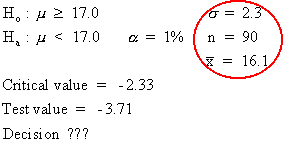### CFA Practice Question

There are 985 practice questions for this topic.

### CFA Practice Question

For the hypothesis test shown below, the decision should be to ______.A. conclude Ha
B. fail to reject H0
C. reject H0

Because the sample data, x-bar 16.1, has a z-score of -3.71 (the test value) and this z-score is in the critical region (critical value is -2.33, the cutoff for the bottom 1% of the normal distribution), the decision is to reject H0. That is, we do not believe this sample data came from a population whose mean is 17 or more. Note that the p-value for an x-bar of 16.1 is 0+.

User Comment
Yurik74 How -2.33 is calculated??? I understand that -3.71 = (16.1-17)/(2.3/sqrt(90))
surjoy Z 0.01 is 2.33
JKiro Yurik74: critical values (rejection points) are determined by the experimenters
VGVG Isn't this a one-tailed test? And for a one-tailed test, the decision rule is:

Reject H0 if z > z-alpha.

In this case, z-score of -3.71 can't be > -2.33
VGVG Never mind, this is a one-tailed test with:
H0: u >= u0, and for this the rejection clause is: z < -z-alpha